# ISRO Scientist or Engineer Mechanical May 2017

Instructions

For the following questions answer them individually

Question 71

# A force P is applied at a distance ‘ X ’ from the end of the beam as shown in the figure. What would be value of ‘ X ’ so that the displacement at ‘A’ is equal to zero?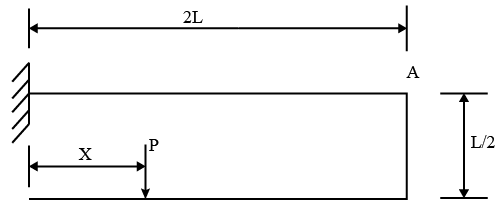Question 72

# An electric heater is sandwiched between two plates each 0.3 m long and 0.1 m wide with a thickness of 30 mm. At steady state condition the heater is maintained at a temperature of $$100^\circ C$$, with a current of 0.25 A and voltage of 200 V. Assume the plates are perfectly insulated at the edges, and the heater is having perfect contact with the plates to give a temperature of $$50^\circ C$$ on the outside of the plate surface. What is the thermal conductivity of the plate material?Question 73

# Knife line or hairline cracking is observed inQuestion 74

# Riser is designed so as toQuestion 75

# A torque T is applied at the free end of a stepped rod of circular cross-sections as shown in the figure. The shear modulus of the material of the rod is G. The expression for diameter ‘d’ to produce an angular twist q at the free end is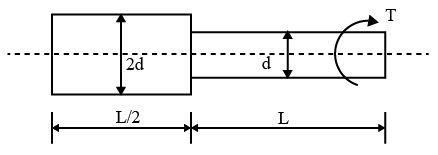Question 76

# A pin jointed uniform rigid rod of weight 981 N and length 1 m is kept horizontally by an external force F as shown in the figure below. The force F is removed suddenly. At the instant of force removal, the magnitude of vertical reaction (in N) developed at the support is close to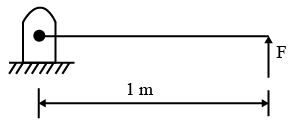Question 77

# The frictional torque transmitted in a conical pivot bearing considering uniform pressure is : (R: radius of shaft,a : semi angle of cone, $$\mu$$ : Coefficient of friction, W: load on bearing)Question 78

# A mass of 2 kg is attached to two identical springs each with stiffness k = 40 kN/m as shown in the figure. Under friction less condition, the natural frequency of the system in Hz is close to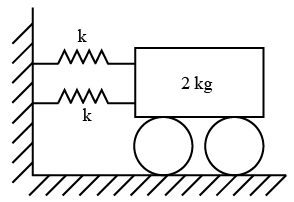Question 79

# A rectangular steel plate is joined to a vertical post using four identical rivets arranged as shown below in the figure. The shear load on the worst loaded rivet (in N) approximately is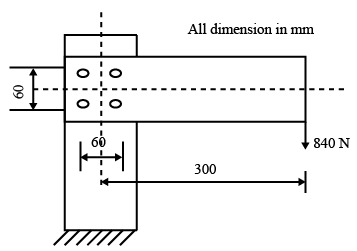Question 80

# Match the following criterion of material failure under biaxial stresses $$\sigma_1$$ and $$\sigma_2$$ and yield stress $$\sigma_y$$ , with their corresponding graphic representations.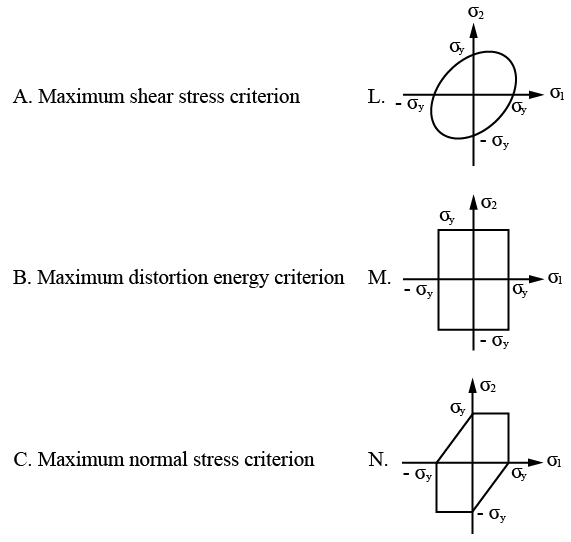OR